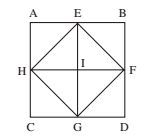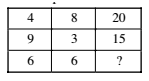# SSC CGL 2018 Practice Test Papers | Reasoning Ability (Day-46)

Dear Aspirants, Here we have given the Important SSC CGL Exam 2018 Practice Test Papers. Candidates those who are preparing for SSC CGL 2018 can practice these questions to get more confidence to Crack SSC CGL 2018 Examination.

[WpProQuiz 3381]

Click “Start Quiz” to attend these Questions and view Explanation

1) How many squares are there in the following figure?a) 6

b) 8

c) 4

d) 5

2) Which of the numbers would be filled in the blank space?a) 22

b) 20

c) 18

d) 24

3) Three out of four are similar in a certain way and form a group. Which one does not belong to the group?

a) DFH

b) TVX

c) NPR

d) XZC

4) Some letters are missing in the following letter series. The missing letters are given in the proper sequence as one of the alternatives among the five given below. Find the correct alternative.

a — b — c — a — b

a) aabc

b) cbcb

c) acba

d) cabc

5) Ramesh began to walk towards North from the point A. He turned to his left after walking 10 m to reach Point B and walked 15 m to reach point C. Then he turned to his right and walked 5 m for point D. Again he turned to his right and walked 15 m to finish in Point E. Now how far he is from his starting point?

a) 10 m

b) 15 m

c) 35 m

d) 45 m

6) How many words in English can be made by using the letters of the word ‘SATELLITE’? Each letter can be used only once and the order of letters should remain the same.

a) 1

b) 2

c) 3

d) More than 3

7) Find out the number that would replace the question mark (?) in each of the following questions

350, 520, 738, ?, 1342

a) 1010

b) 980

c) 1100

d) 950

8) If by looking in a mirror it appears that it is 8: 30 in the clock, what is the real time?

a) 4: 30

b) 6: 30

c) 3: 30

d) 6: 10

9) If the 1st and 6th letters of the word ‘ARITHMETIC ’ are interchanged, also 2nd and 7th letters, and so on, which of the following would be the 4th letter from your right?

a) A

b) R

c) M

d) C

10) Statement:

K > M ≤ R; T < U = W; W > N > O = M

Conclusion:

1. T > N
2. R < W

a) Only I follow

b) Only II follows

c) Both I and II

d) Neither I nor II follows

(i) ABDC (ii) AEIH (iii) EBFI (iv) HIGC (v) IFDG (vi) HEFG

8 × 2 + 4 = 20

2 × 3 + 9 = 15

2 × 6 + 6 = 18

D + 2 = F;              F + 2 = H

T + 2 = V;              V + 2 = X

N + 2 = P;             P + 2 = R

X + 2 = Z;              Z + 3 = C

acb/acb/acb is formed in which acb is repeated thrice.Words formed: SALE, SIT, ELITE, TELL etc.,

350         520          738            1010            1342

73+7       83+8         93+9           103+10         113+11

When the hour hand of the clock is between 3 and 4, it will appear to between 8 and 9 in the mirror and when the minute hand is on 6 it will appear in the mirror on 6. Thus when it is actually 3: 30 in a clock it will appear 8: 30 in the mirror.

After interchanging, the word formed is METICARITH

Fourth letter from right = R